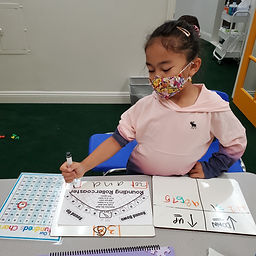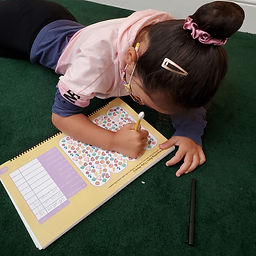## Ms. Raejean

### Target 1​

###### Lesson Type:

New

Number Operation

:

Integer Composition

Use place-value understandings to round whole numbers to the nearest ten or 100.

###### 1:

Use understanding of how to round two-digit numbers and expand the strategy to three-digit numbers.

###### 2:

Understand that rounding identifies the multiple of 10 or 100 that is closest to the given number.

###### 3:

Understand that the previous place (one place smaller) is the key digit to evaluate to determine whether a number is rounded up or down.

3rd

###### Vocabulary:

Rounding

Activities:

• The term "rounding" was introduced. We round to make numbers "friendly" and easier to use. We did a quick review of place value (ones, tens, and hundreds place). Students also identified the multiples of 10 (10, 20, 30, 40, 50, 60, 70, 80, 90, 100).
• We focused on rounding 2-digit numbers to the nearest ten. Students referred to a hundreds chart to help them determine which 2 tens the given number was in between. (Ex. 34 is in between 30 and 40) Students identified the ten that the given number was closer to. (Ex. 34 is closer to 30. It's 4 away from 30 and 6 away from 40; therefore, 34 rounded to the nearest ten is 30.)
• We also worked on using place value to help round the 2-digit numbers. We looked at the number in the ones place to see if we should round up or down. (Ex. 34 has a 4 in the ones place, and we rounded down. 26 has a 6 in the ones place, and we rounded up.) The "rounding rhyme" was introduced. ~ Underline the digit, look next door. If it's 5 or higher, add one more. If it's 4 or lower, just ignore. Students completed the "rounding rollercoaster."### Home Exploration

###### Guiding Questions:## Absent Students:

### Target 2

:

###### 1:

Use tally marks to collect and track quantitative data.

###### 2:

Looking at a picture where information is chaotic or spread out, count to gather data to answer questions like, how many are there? Are there more ___ or ___? How many more ___ are there than ___?

2nd

###### Vocabulary:

Logic Reasoning, Interpret, Quantity, Tally Marks

Activities:

• Students counted the amount of various items in a given picture. Students used tally marks to keep track of the data.### Home Exploration

###### Guiding Questions:### Target 3

:

###### Vocabulary:

Activities:### Home Exploration